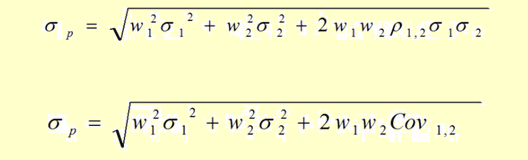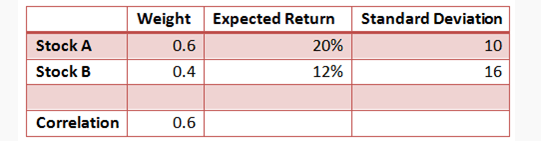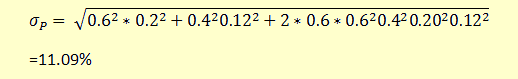# Variance and Standard Deviation of a Portfolio

We learned about how to calculate the standard deviation of a single asset. Let’s now look at how to calculate the standard deviation of a portfolio with two or more assets.

The returns of the portfolio were simply the weighted average of returns of all assets in the portfolio. However, the calculation of the risk/standard deviation is not the same. While calculating the variance, we also need to consider the covariance between the assets in the portfolio. If the assets are perfectly correlated, then the simple weighted average of variances will work. However, when we have to account for the covariance, the equation will change.

Covariance reflects the degree to which two securities vary or change together, and is represented as Cov (Ri,Rj). The problem with covariance is that it has no units, and is difficult to compare across assets. Using covariance, we can calculate the correlation between the assets using the following formula:Correlation

After incorporating covariance, the standard deviation of a two-asset portfolio can be calculated as follows:Standard Deviation of a Two Asset Portfolio

In general as the correlation reduces, the risk of the portfolio reduces due to the diversification benefits. Two assets that are perfectly negatively correlated provide the maximum diversification benefit and hence minimize the risk.

Example

Assume we have a portfolio with the following details:The standard deviation can be calculated as follows:This portfolio has an expected return of 16.80% and a portfolio risk of 11.09%.

Get our Data Science for Finance Bundle for just $29$51.